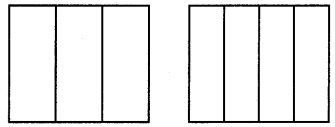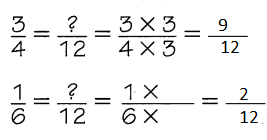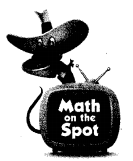# Texas Go Math Grade 5 Lesson 5.4 Answer Key Common Denominators and Equivalent Fractions

Refer to our Texas Go Math Grade 5 Answer Key Pdf to score good marks in the exams. Test yourself by practicing the problems from Texas Go Math Grade 5 Lesson 5.4 Answer Key Common Denominators and Equivalent Fractions.

## Texas Go Math Grade 5 Lesson 5.4 Answer Key Common Denominators and Equivalent Fractions

Unlock the Problem

Sarah planted two 1-acre gardens. One had 3 sections of flowers and the other had 4 sections of flowers. She plans to divide both gardens into more sections so that they have the same number of equal-sized sections. How many sections will each garden have?

You can use a common denominator or a common multiple of two or more denominators to write fractions that name the same part of a whole.

Find the common denominator.
ThinkDivide each $$\frac{1}{3}$$ into fourths and divide each $$\frac{1}{4}$$ into thirds, each of the wholes will be divided into the same size parts, twelfths.

Record

• Multiply the denominators to find a common denominator.
A common denominator of $$\frac{1}{3}$$ and $$\frac{1}{4}$$ is __________.
• Write and as equivalent fractions using the common denominator.
$$\frac{1}{3}$$ = ___________ $$\frac{1}{4}$$ = ____________

So, both gardens will have ___________ sections.

• Multiply the denominators to find a common denominator.
A common denominator of $$\frac{1}{3}$$ and $$\frac{1}{4}$$ is 12
• Write and as equivalent fractions using the common denominator.
$$\frac{1}{3}$$ =$$\frac{4}{12}$$
$$\frac{1}{4}$$ = $$\frac{3}{12}$$

So, both gardens will have12 sections.

Find the least common denominator of $$\frac{3}{4}$$ and $$\frac{1}{6}$$.

List nonzero multiples of the denominators.
Find the least common multiple.
Multiplesof 4: ___________________
Multiples of 6: ___________________
So, the least common denominator of $$\frac{3}{4}$$ and $$\frac{1}{6}$$ is _______________.
List nonzero multiples of the denominators.
Find the least common multiple.
Multiples of 4:    2 , 4
Multiples of 6:   2, 3, 6
So, the least common denominator of $$\frac{3}{4}$$ and $$\frac{1}{6}$$ is 12.

Use the least common denominator to write equivalent fractions.$$\frac{3}{4}$$ can be rewritten as ______________ and $$\frac{1}{6}$$ can be rewritten as ____________.$$\frac{3}{4}$$ can be rewritten as $$\frac{9}{12}$$ and $$\frac{1}{6}$$ can be rewritten as $$\frac{2}{12}$$ .

Share and Show

Find a common denominator of $$\frac{1}{6}$$ and $$\frac{1}{9}$$. Rewrite the pair of fractions using the common denominator.

• Multiply the denominators.
A common denominator of $$\frac{1}{6}$$ and $$\frac{1}{9}$$ is ___________.
• Rewrite the pair of fractions using the common denominator.
$$\frac{1}{6}$$ = ___________ $$\frac{1}{9}$$ = ____________

• Multiply the denominators.
A common denominator of $$\frac{1}{6}$$ and $$\frac{1}{9}$$ is 18.
• Rewrite the pair of fractions using the common denominator.
$$\frac{1}{6}$$ = $$\frac{3}{18}$$
$$\frac{1}{9}$$ = $$\frac{2}{18}$$

Explanation:
By using A common denominator written an equivalent fraction for each fraction

Math Talk
Mathematical Processes

Explain two methods for finding a common denominator of two fractions.
Prime Factorization Method and Division method

Use a common denominator to write an equivalent fraction for each fraction.

Question 2.
$$\frac{1}{3}$$, $$\frac{1}{5}$$
common denominator: ___________

• Multiply the denominators.
A common denominator of $$\frac{1}{3}$$ and $$\frac{1}{5}$$ is 15.
• Rewrite the pair of fractions using the common denominator.
$$\frac{1}{3}$$ = $$\frac{5}{15}$$
$$\frac{1}{5}$$ = $$\frac{3}{15}$$

Explanation:
By using A common denominator written an equivalent fraction for each fraction

Question 3.
$$\frac{2}{3}$$ , $$\frac{5}{9}$$
common denominator: ___________

• Multiply the denominators.
A common denominator of $$\frac{2}{3}$$ and $$\frac{5}{9}$$ is 9.
• Rewrite the pair of fractions using the common denominator.
$$\frac{2}{3}$$ = $$\frac{6}{9}$$
$$\frac{5}{9}$$ = $$\frac{5}{9}$$

Explanation:
By using A common denominator write an equivalent fraction for each fraction

$$\frac{2}{9}$$, $$\frac{1}{15}$$
common denominator: _____________

• Multiply the denominators.
A common denominator of $$\frac{2}{9}$$ and $$\frac{1}{15}$$is 15.
• Rewrite the pair of fractions using the common denominator.
$$\frac{2}{9}$$ = $$\frac{10}{45}$$
$$\frac{1}{15}$$ = $$\frac{3}{45}$$

Explanation:
By using A common denominator written an equivalent fraction for each fraction

Use the least common denominator to write an equivalent fraction for each fraction.

Question 5.
$$\frac{1}{4}$$, $$\frac{3}{8}$$
least common denominator: _____________

Explanation:
a “Denominator” is the bottom number of a fraction. a “Common Denominator” is when the bottom number is the same for the fractions. the “Least Common Denominator” is the smallest number that can be used for all denominators of the fractions. It makes it easy to add and subtract the fractions.

Question 6.
$$\frac{11}{12}$$, $$\frac{5}{8}$$
least common denominator: _____________
Explanation:
a “Denominator” is the bottom number of a fraction. a “Common Denominator” is when the bottom number is the same for the fractions. the “Least Common Denominator” is the smallest number that can be used for all denominators of the fractions. It makes it easy to add and subtract the fractions.

Question 7.
$$\frac{4}{5}$$, $$\frac{1}{6}$$
least common denominator: _____________
Explanation:
a “Denominator” is the bottom number of a fraction. a “Common Denominator” is when the bottom number is the same for the fractions. the “Least Common Denominator” is the smallest number that can be used for all denominators of the fractions. It makes it easy to add and subtract the fractions.

Problem Solving

Practice: Copy and Solve Use the least common denominator to write an equivalent fraction for each fraction.

Question 8.
$$\frac{1}{6}$$, $$\frac{4}{9}$$
$$\frac{1}{6}$$ = $$\frac{3}{18}$$
$$\frac{4}{9}$$ = $$\frac{8}{18}$$
Explanation:

• A common denominator of $$\frac{1}{6}$$, $$\frac{4}{9}$$ is 18.
• Rewrite the pair of fractions using the common denominator.
$$\frac{1}{6}$$= $$\frac{3}{18}$$
$$\frac{4}{9}$$, = $$\frac{8}{18}$$

Question 9.
$$\frac{7}{9}$$, $$\frac{8}{27}$$

$$\frac{7}{9}$$ = $$\frac{21}{27}$$
$$\frac{8}{27}$$= $$\frac{8}{27}$$
Explanation:

• A common denominator of $$\frac{7}{9}$$, $$\frac{8}{27}$$ is 27.
• Rewrite the pair of fractions using the common denominator.
$$\frac{7}{9}$$ = $$\frac{21}{27}$$
$$\frac{8}{27}$$= $$\frac{8}{27}$$

$$\frac{7}{10}$$, $$\frac{3}{8}$$

$$\frac{7}{10}$$ = $$\frac{28}{40}$$
$$\frac{3}{8}$$= $$\frac{15}{40}$$
Explanation:

• A common denominator of $$\frac{7}{10}$$, $$\frac{3}{8}$$ is 40.
• Rewrite the pair of fractions using the common denominator.
$$\frac{7}{10}$$ = $$\frac{28}{40}$$
$$\frac{3}{8}$$= $$\frac{15}{40}$$

Question 11.
$$\frac{1}{3}$$, $$\frac{5}{11}$$

$$\frac{1}{3}$$ = $$\frac{11}{33}$$
$$\frac{5}{11}$$= $$\frac{15}{33}$$
Explanation:

• A common denominator of $$\frac{1}{3}$$, $$\frac{5}{11}$$ is 33.
• Rewrite the pair of fractions using the common denominator.
$$\frac{1}{3}$$ = $$\frac{11}{33}$$
$$\frac{5}{11}$$= $$\frac{15}{33}$$

H.O.T. Algebra Write the unknown number for each.

Question 12.
$$\frac{1}{5}$$, $$\frac{1}{8}$$
least common denominator:= _____________
Explanation:
Found the unknown number in each expression

Question 13.
$$\frac{2}{5}$$, $$\frac{1}{}$$
least common denominator: 15= _____________
Answer: $$\frac{1}{3}$$
Explanation:
Found the unknown number in each expression

Question 14.
$$\frac{3}{}$$, $$\frac{5}{6}$$
least common denominator: 42= _____________
Answer: $$\frac{3}{7}$$
Explanation:
Found the unknown number in each expression

Question 15.
What does a common denominator of two fractions represent? Explain.
Explanation:
common denominator of two fractions represent multiples of the fraction

Unlock the Problem

Question 16.
Katie made two pies for the bake sale. One was cut into three equal slices and the other into 5 equal slices. She will continue to cut the pies so each one has the same number of equal-sized slices. What is the least number of equal-sized slices each pie could have?
a. What information are you given?
The information is about pie making into slices and sharing them to equal shares

b. What problem are you being asked to solve?
least number of equal-sized slices each pie had.

c. When Katie cuts the pies more, can she cut each pie the same number of times and have all the slices the same size? Explain.
When Katie cuts the slices to 3 times the slices as 5
Katie cuts the pies more,  she  can’t cut each pie the same number of times and have all the slices doesn’t have the same size

d. Use the diagram to show the steps you use to solve the problem.Step 1:
The first one is sliced once again so it is again made 2 share
Step 2:
In complete the first pie also have 5 parts

e. Complete the sentences.
The least common denominator of $$\frac{1}{3}$$ and $$\frac{1}{5}$$ is __________.
Katie can cut each piece of the first pie into ________ and each piece of the second pie into _________.
That means that Katie can cut each pie into pieces that are ________ of the whole pie.
The least common denominator of $$\frac{1}{3}$$ and $$\frac{1}{5}$$ is 15
Katie can cut each piece of the first pie into $$\frac{5}{15}$$ and each piece of the second pie into $$\frac{3}{15}$$
That means that Katie can cut each pie into pieces that are $$\frac{8}{15}$$ of the whole pie.

Question 17.
H.O.T. Multi-Step Arnold had three pieces of different color string all the same length. Arnold cut the blue string into 2 equal lengths. He cut the red string into 3 equal-size lengths, and the green string into 6 equal-size lengths. He needs to cut the string so each color has the same number of equal-size lengths. What is the least number of equal-sized lengths each color string could have?Answer: 12 each color string could have
Explanation:
Arnold had three pieces of different color string all the same length.
Arnold cut the blue string into 2 equal lengths.
He cut the red string into 3 equal-size lengths,
and the green string into 6 equal-size lengths.
He needs to cut the string so each color has the same number of equal-size lengths
12 the least number of equal-sized lengths each color string could have.

Question 18.
Reasoning Magara entered the fractions $$\frac{1}{4}$$ and $$\frac{7}{}$$ into a computer program. The computer used the least common denominator to rename the fractions as $$\frac{5}{20}$$ and $$\frac{14}{20}$$. What is the unknown denominator?
(A) 20
(B) 8
(C) 12
(D) 10
Explanation:
Magara entered the fractions $$\frac{1}{4}$$ and $$\frac{7}{}$$ into a computer program.
The computer used the least common denominator to rename the fractions as
$$\frac{5}{20}$$ and $$\frac{14}{20}$$. The unknown denominator is 20

Alejandro wants to use the least common denominator to write equivalent fractions for $$\frac{3}{7}$$ and $$\frac{4}{5}$$. He rewrites the fractions as $$\frac{15}{35}$$ and $$\frac{20}{35}$$. How should he change his answer?
(A) The numerators are correct, but the denominators should be 7.
(B) $$\frac{20}{35}$$ is correct, but $$\frac{15}{35}$$ should be $$\frac{21}{25}$$.
(C) $$\frac{15}{35}$$ is correct, but $$\frac{20}{35}$$ should be $$\frac{28}{35}$$.
(D) The denominators are correct, but both numerators should be 12.
Explanation:
Alejandro wants to use the least common denominator to write equivalent fractions for
$$\frac{3}{7}$$ and $$\frac{4}{5}$$.
He rewrites the fractions as
$$\frac{15}{35}$$ and $$\frac{20}{35}$$. he changes the answer to
$$\frac{15}{35}$$ is correct, but $$\frac{20}{35}$$ should be $$\frac{28}{35}$$.

Question 20.
Multi-Step Aiesha and her mom are cutting two sandwiches into smaller bite-size pieces. They cut the first sandwich in four equal sized pieces. They cut the second sandwich into six equal-sized pieces. However, they want an equal number of pieces from each sandwich. What is the least number of pieces they could cut from each sandwich?
(A) 4
(B) 6
(C) 10
(D) 12
Explanation:
Aiesha and her mom are cutting two sandwiches into smaller bite-size pieces.
They cut the first sandwich in four equal sized pieces.
They cut the second sandwich into six equal-sized pieces.
However, they want an equal number of pieces from each sandwich.
10 is the least number of pieces they could cut from each sandwich

Texas Test Prep

Question 21.
Which fractions use the least common denominator and are equivalent to $$\frac{5}{8}$$ and $$\frac{7}{10}$$ ?
(A) $$\frac{10}{40}$$ and $$\frac{14}{40}$$
(B) $$\frac{25}{80}$$ and $$\frac{21}{80}$$
(C) $$\frac{25}{40}$$ and $$\frac{28}{40}$$
(D) $$\frac{50}{80}$$ and $$\frac{56}{80}$$
Explanation:
least common denominator and are equivalent to $$\frac{5}{8}$$ and $$\frac{7}{10}$$
is $$\frac{25}{40}$$ and $$\frac{28}{40}$$

### Texas Go Math Grade 5 Lesson 5.4 Homework and Practice Answer Key

Use the least common denominator to write an equivalent fraction for each fraction.

Question 1.
$$\frac{1}{10}$$, $$\frac{1}{5}$$ ___________
$$\frac{1}{10}$$ = $$\frac{1}{10}$$
$$\frac{1}{5}$$= $$\frac{2}{10}$$

Explanation:

• A common denominator of $$\frac{1}{10}$$, $$\frac{1}{5}$$ is 10
• Rewrite the pair of fractions using the common denominator.
$$\frac{1}{10}$$ = $$\frac{1}{10}$$
$$\frac{1}{5}$$= $$\frac{2}{10}$$

Question 2.
$$\frac{1}{3}$$, $$\frac{2}{9}$$ ___________
$$\frac{1}{3}$$ = $$\frac{3}{9}$$
$$\frac{2}{9}$$= $$\frac{2}{9}$$

Explanation:

• A common denominator of $$\frac{1}{3}$$, $$\frac{2}{9}$$ is 9
• Rewrite the pair of fractions using the common denominator.
$$\frac{1}{3}$$ = $$\frac{3}{9}$$
$$\frac{2}{9}$$= $$\frac{2}{9}$$

Question 3.
$$\frac{1}{6}$$, $$\frac{2}{4}$$ ___________
$$\frac{1}{6}$$ = $$\frac{2}{12}$$
$$\frac{2}{4}$$= $$\frac{6}{12}$$

Explanation:

• A denominator of $$\frac{1}{6}$$, $$\frac{2}{4}$$ is 12
• Rewrite the pair of fractions using the common denominator.
$$\frac{1}{6}$$ = $$\frac{2}{12}$$
$$\frac{2}{4}$$= $$\frac{6}{12}$$

Question 4.
$$\frac{2}{3}$$, $$\frac{1}{2}$$ ___________
$$\frac{2}{3}$$ = $$\frac{4}{6}$$
$$\frac{1}{2}$$= $$\frac{3}{6}$$

Explanation:

• A common denominator of $$\frac{2}{3}$$, $$\frac{1}{2}$$ is 6.
• Rewrite the pair of fractions using the common denominator.
$$\frac{2}{3}$$ = $$\frac{4}{6}$$
$$\frac{1}{2}$$= $$\frac{3}{6}$$

Question 5.
$$\frac{3}{4}$$, $$\frac{3}{8}$$ ___________
$$\frac{3}{4}$$ = $$\frac{6}{8}$$
$$\frac{3}{8}$$= $$\frac{3}{8}$$

Explanation:

• A common denominator of $$\frac{3}{4}$$, $$\frac{3}{8}$$ is8
• Rewrite the pair of fractions using the common denominator.
$$\frac{3}{4}$$ = $$\frac{6}{8}$$
$$\frac{3}{8}$$= $$\frac{3}{8}$$

Question 6.
$$\frac{11}{12}$$, $$\frac{1}{6}$$ ___________
$$\frac{11}{12}$$ = $$\frac{11}{12}$$
$$\frac{1}{6}$$= $$\frac{2}{12}$$

Explanation:

• A common denominator of $$\frac{11}{12}$$, $$\frac{1}{6}$$ is 12.
• Rewrite the pair of fractions using the common denominator.
$$\frac{11}{12}$$ = $$\frac{11}{12}$$
$$\frac{1}{6}$$= $$\frac{2}{12}$$

Question 7.
$$\frac{1}{2}$$, $$\frac{2}{5}$$ ___________
$$\frac{1}{2}$$ = $$\frac{5}{10}$$
$$\frac{2}{5}$$= $$\frac{4}{10}$$

Explanation:

• A common denominator of $$\frac{1}{2}$$, $$\frac{2}{5}$$ is 10
• Rewrite the pair of fractions using the common denominator.
$$\frac{1}{2}$$ = $$\frac{5}{10}$$
$$\frac{2}{5}$$= $$\frac{4}{10}$$

Question 8.
$$\frac{5}{7}$$, $$\frac{3}{5}$$ ___________
$$\frac{5}{7}$$/ = $$\frac{25}{35}[latex] [latex]\frac{3}{5}$$= $$\frac{21}{35}$$

Explanation:

• A common denominator of $$\frac{5}{7}$$, $$\frac{3}{5}$$ is 35
• Rewrite the pair of fractions using the common denominator.
$$\frac{5}{7}$$/ = $$\frac{25}{35}[latex] [latex]\frac{3}{5}$$= $$\frac{21}{35}$$

Go Math Common Denominators and Equivalent Fractions Lesson 5.4 Question 9.
$$\frac{1}{4}$$, $$\frac{3}{16}$$ ___________
$$\frac{1}{4}$$ = $$\frac{4}{16}$$
$$\frac{3}{16}$$= $$\frac{3}{16}$$

Explanation:

• A common denominator of $$\frac{1}{4}$$, $$\frac{3}{16}$$ is 16
• Rewrite the pair of fractions using the common denominator.
$$\frac{1}{4}$$ = $$\frac{4}{16}$$
$$\frac{3}{16}$$= $$\frac{3}{16}$$

Question 10.
$$\frac{2}{5}$$, $$\frac{3}{4}$$ ___________
$$\frac{2}{5}$$ = $$\frac{8}{20}$$
$$\frac{3}{4}$$= $$\frac{15}{20}$$

Explanation:

• A common denominator of $$\frac{2}{5}$$, $$\frac{3}{4}$$ is 20
• Rewrite the pair of fractions using the common denominator.
$$\frac{2}{5}$$ = $$\frac{8}{20}$$
$$\frac{3}{4}$$= $$\frac{15}{20}$$

Question 11.
$$\frac{2}{15}$$, $$\frac{5}{6}$$ ___________
$$\frac{2}{15}$$ = $$\frac{4}{30}$$
$$\frac{5}{6}$$= $$\frac{25}{30}$$

Explanation:

• A common denominator of $$\frac{2}{15}$$, $$\frac{5}{6}$$ is 30
• Rewrite the pair of fractions using the common denominator.
$$\frac{2}{15}$$ = $$\frac{4}{30}$$
$$\frac{5}{6}$$= $$\frac{25}{30}$$

Question 12.
$$\frac{7}{8}$$, $$\frac{1}{2}$$ ___________
$$\frac{7}{8}$$ = $$\frac{7}{8}$$
$$\frac{1}{2}$$= $$\frac{4}{8}$$

Explanation:

• A common denominator of $$\frac{7}{8}$$, $$\frac{1}{2}$$ is 8.
• Rewrite the pair of fractions using the common denominator.
$$\frac{7}{8}$$ = $$\frac{7}{8}$$
$$\frac{1}{2}$$= $$\frac{4}{8}$$

Write the unknown number for each.

Question 13.
$$\frac{2}{3}$$, $$\frac{1}{6}$$
least common denominator:= _____________
Explanation:
Found the unknown number in each expression

Question 14.
$$\frac{1}{8}$$, $$\frac{2}{}$$
least common denominator: 24= _____________
Answer: $$\frac{2}{3}$$
Explanation:
Found the unknown number in each expression

Question 15.
$$\frac{1}{}$$, $$\frac{2}{7}$$
least common denominator: 21= ____________
Answer: $$\frac{1}{3}$$
Explanation:
Found the unknown number in each expression

Problem Solving

Question 16.
Dana bought two same-sized posterboards. She cut the posterboards into equal-sized pieces to make placemats for her dinner guests. She cut the first posterboard into 5 pieces and the second posterboard into 2 pieces. She will continue to cut the pieces of posterboard so that each one is divided into the same number of equal-sized pieces. What is the least number of equal-sized pieces each posterboard could have?
Explanation:
least number of equal-sized pieces each posterboard could have is 10

Question 17.
A recipe for homemade goop calls for $$\frac{1}{4}$$ cup of cornstarch and $$\frac{1}{8}$$ cup of glue. Find the least common denominator of the fractions used in the recipe.
Explanation:
least common denominator of the fractions used in the recipe is 8

Lesson Check

Question 18.
How can you find the least common denominator for $$\frac{1}{8}$$ and $$\frac{2}{9}$$.
(A) Multiply 8 and 9.
(C) Multiply each number by 2.
(D) Add 2 to 8 and 1 to 9.
Explanation:
a “Denominator” is the bottom number of a fraction. a “Common Denominator” is when the bottom number is the same for the fractions. the “Least Common Denominator” is the smallest number that can be used for all denominators of the fractions. It makes it easy to add and subtract the fractions.

Go Math Lesson 5.4 Homework Answer Key Question 19.
If the least common denominator for $$\frac{1}{}$$ and $$\frac{5}{12}$$ is 12, which of the following could not be the unknown denominator?
(A) 2
(B) 3
(C) 4
(D) 5
Explanation:
Remaining are the multiples of 12

Question 20.
Which fractions use the least common denominator and are equivalent to $$\frac{3}{10}$$ and $$\frac{1}{6}$$?
(A) $$\frac{18}{60}$$ and $$\frac{10}{60}$$
(B) $$\frac{30}{60}$$ and $$\frac{10}{60}$$
(C) $$\frac{10}{30}$$ and $$\frac{18}{30}$$
(D) $$\frac{5}{30}$$ and $$\frac{9}{30}$$
Explanation:
The least common denominator and are equivalent to $$\frac{3}{10}$$ and $$\frac{1}{6}$$ is $$\frac{5}{30}$$ and $$\frac{9}{30}$$

Question 21.
Lindsay writes two fractions with a least common denominator of 36. Which fractions does Lindsay write?
(A) $$\frac{2}{3}$$, $$\frac{5}{12}$$
(B) $$\frac{2}{9}$$, $$\frac{1}{12}$$
(C) $$\frac{3}{8}$$, $$\frac{7}{72}$$
(D) $$\frac{1}{8}$$, $$\frac{5}{36}$$
Explanation:
Lindsay writes two fractions with a least common denominator of 36
Lindsay fraction is $$\frac{2}{9}$$, $$\frac{1}{12}$$

Question 22.
Multi-Step An archeologist marks off two equal-sized sites for excavation. She uses a grid system to divide each square site into sections. One square has 8 sections. The other square has 6 sections. She plans to divide both squares into more sections so that they have the same number of equal-sized sections. How many sections will each square have?
(A) 14
(B) 8
(C) 24 .
(D) 36
Explanation:
An archeologist marks off two equal-sized sites for excavation.
She uses a grid system to divide each square site into sections.
One square has 8 sections. The other square has 6 sections.
She plans to divide both squares into more sections so that they have the same number of equal-sized sections.
24sections will each square have

Question 23.
Multi-Step Mr. Nickelson tells the class that they double the least common denominator for $$\frac{1}{2}$$, $$\frac{3}{5}$$, and $$\frac{9}{15}$$ to find the number of the day. Which number is the number of the day?
(A) 30
(B) 15
(C) 60
(D) 32
Explanation: least common denominator for $$\frac{1}{2}$$, $$\frac{3}{5}$$, and $$\frac{9}{15}$$ = 30